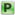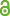Hauptmenü
• Autor
• Yang, Daodao
• TitelExtreme values of derivatives of the Riemann zeta function
• Datei
• DOI10.1112/mtk.12130
• Erschienen inMathematika
• Band68
• Erscheinungsjahr2022
• Heft2
• Seiten486-510
• LicenceCC BY-NC-ND 4.0
• ISSN2041-7942
• Zugriffsrechte• AbstractIt is proved that if 𝑇 is sufficiently large, then uniformly for all positive integers 𝓁 ⩽ (log 𝑇)∕(log2 𝑇), we have $$\max_{𝑇⩽𝑡⩽2𝑇} 𝜁^{(l)} 1 + 𝑖𝑡| ⩾ 𝑒^𝛾 ⋅ l^l ⋅ (l + 1)^{−(l+1)} ⋅ ( log_2𝑇 −log_3𝑇 +𝑂(1)^{l+1}$$ , where 𝛾 is the Euler constant. We also establish lower bounds for maximum of $$|𝜁^{(l)}(𝜎 + 𝑖𝑡)|$$ when 𝓁 ∈ ℕ and 𝜎 ∈ [1∕2, 1) are fixed. MSC (202 0 ) 11M06 (primary)